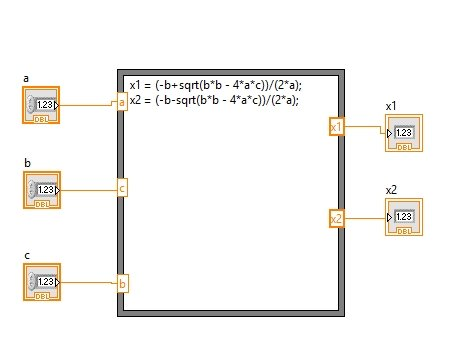# Quadratic root calculation in LabView : tutorial 26

In this tutorial I will tell you how to calculation roots of a quadratic equation using LabView. Quadratic roots calculation is one of the most easiest but hectic task near me. In this tutorial I will help you design a VI that will take input as the coefficients of a quadratic equation and at the output; it will return you with the roots of the equation you gave as input. At the end of the tutorial you are provided with an exercise to do it by yourself, and in the next tutorials I will assume that you have done those exercises and I will not explain the concept regarding them.

### Quadratic root calculation in LabView example

Figure 1: Numeric control placement

Figure 2: Input controls

Figure 3: Numeric indicator placement

Figure 4: Output indicator

Figure 5: Formula node placement

Figure 8: Inputs and outputs

Figure 9: Connections

Figure 10: Formula code

• If you are not familiar with any of the command in LabView simple open the built in help window of LabView see the detailed description of the command or block about which you want to know.
• The nodes you created on the input and output side of the formula node will store the value of the controls you connected to them or for the output case they will display the value from the formula node to the output indicator.
• But, where will it store the value from the input control?? We have to name the node with a specific variable in which it will store the value from the input control and will use that dummy variable inside the formula node.
• Similar is the case with the output nodes, all the values assigned to any dummy variable inside the formula node i.e. x1 and x2 in our case will have one output node assigned to it.
• No of output nodes must be equal to the number of assignment operator used inside the formula node and we have to name output nodes too according to the dummy variable inside the formula node as shown in the figure below,Figure 11: Naming of the node.

Figure 12: Output of the VI

• The VI we designed here will calculate only the real roots of the quadratic equation

Exercise:

• Use the knowledge you have gained so far and try to generalize the above VI for finding out imaginary roots too.

<<Previous tutorial                                              Next tutorial>>